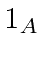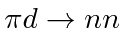## Interchange Symmetry for States with Identical Particles

If we are combining the angular momentum from two identical particles, like two electrons in an atom, we will be interested in the symmetry under interchange of the angular momentum state. Lets use the combination of two spinparticles as an example. We know that we get total spin states ofand. Thestate is called a triplet because there are three states with differentvalues. Thestate is called a singlet.The triplet state is symmetric under interchange. The highest total angular momentum state,, will always be symmetric under interchange.We can see this by looking at the higheststate,. To get the maximum, both spins to have the maximumcomponent. So the product state has just one term and it is symmetric under interchange, in this case,When we lower this state with the (symmetric) lowering operator, the result remains symmetric under interchange. To make the next highest state, with two terms, we must choose a state orthogonal to the symmetric state and this will always be antisymmetric.

In fact, for identical particles, the symmetry of the angular momentum wave function will alternate, beginning with a symmetric state for the maximum total angular momentum. For example, if we add two spin 2 states together, the resulting states are:,,,and. In the language of group theory, when we take the direct product of two representations of the the SU(2) group we get:where the numbers are the number of states in the multiplet.

* Example: Two electrons in a P state.*
* Example: The parity of the pion from.*

Jim Branson 2013-04-22